# ALPHY5: THERMAL PROPERTIES OF MATTER

##### This unit explains Thermal Properties of Matter

Thermometry.

Thermometry is the science and practice of temperature measurement. Any measurable change in a thermometric probe (e.g. the dilatation of a liquid in a capillary tube, variation of electrical resistance of a conductor, of refractive index of a transparent material, and so on) can be used to mark temperature levels, that should later be calibrated against an internationally agreed unit if the measure is to be related to other thermodynamic variables (if the measure is only needed to establish an ordering in thermal levels, no calibration is required).

Thermometry is sometimes split in meteorological studies in two subfields: contact thermometry and non-contact thermometry. As there can never be complete thermal uniformity at large, thermometry is always associated to a heat transfer problem with some space-time coordinates of measurement, given rise to time-series plots and temperature maps (profiles, if one-dimensional).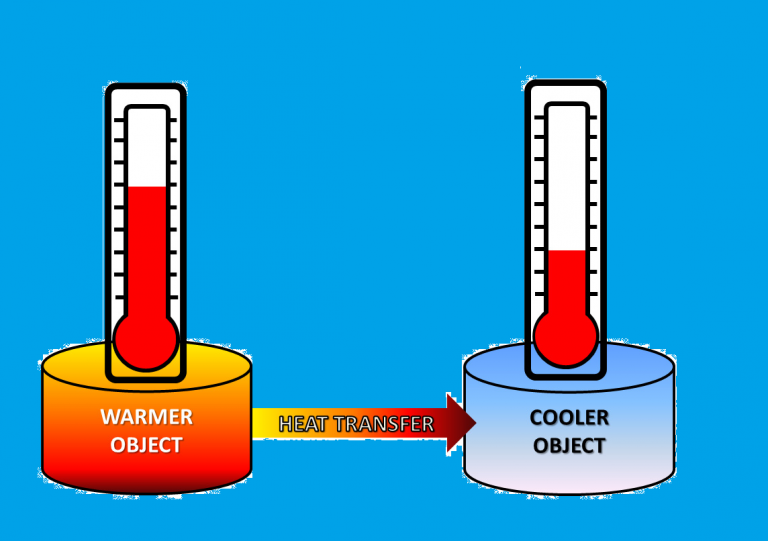Temperature is one of the most measured physical parameters in science and technology; typically for process thermal monitoring and control. But thermometry is not only applied to measuring temperatures for thermal control, but as an indirect measure of many material properties like thermal capacities and enthalpy changes (by thermal analysis), relative humidity (by means of wet bulb or dew-point temperatures), thermo-optical properties and other radiation characteristics (e.g. for the radiation budget of a planet), etc.

Temperature regulation, thermostatic baths, can be based on efficient thermal buffering (e.g. phase change devices), or on controlled heat transfer from a source or to a sink. A thermostat is a device that maintains a system at a constant temperature, issuing a control signal when out of limits. A common thermostat consists of a bimetallic strip that bends as it expands when overheating, switching of the electrical power supply.

Temperature regulation in mammals and birds (homoeothermic animals) is based on the hypothalamus (a neural control centre in the base of the brain also dealing with hunger, thirst, satiety, and other autonomic functions), where some neurons discharge at a rate proportional to absolute temperature, sending nerve signals and hormones to initiate shivering, sweating or panting, and controlling blood flow to limbs and skin to increase or decrease heat losses. In humans, the hypothalamus tries to regulate body temperature to 37±1 ºC under normal circumstances, but allows it to rise to 40 ºC during intensive exercise (to accelerate metabolism) and during infections (to better fight bacteria); there are even some dessert antelopes that allow their body temperature to rise to 45 ºC to save transpiration, while keeping their brains below 40 ºC by panting. Thermal comfort depends a lot on ambient temperature, wind and humidity, but also on subjects (e.g. clothing habits) and other interactions (type of activity, previous accommodation). An abnormally low body temperature, either naturally caused by a cold environment or by disease, or artificially performed to help in heart and brain surgery, is cold hypothermia.

Ambient temperature in meteorology (also named surface temperature) is measured using a thermometer under shelter from direct sun radiation, but well ventilated, placed some 1.5 m above clear ground.

Temperature metrology

Metrology is the science of measurement. Measuring is comparing a physical magnitude with an agreed unit from that magnitude. The result of measuring has three elements: the unit used, and the two multiples or divisions of the unit between which the sample sits; e.g. the mass of a given book is between 1.23 and 1.24 kg (‘1.23’, ‘1.24’, and ‘kg’); equivalently, the three elements may be: the unit, the best estimate value, and the uncertainty. However, it is customary to implicitly assume that the uncertainty is just half the value of the last significant digit quoted in the value, what is a handy practical simplification, but can be pedagogically misleading (how many university students give nonsense results like 1234.56789 K for an adiabatic combustion temperature, for instance).

The seven basic magnitudes in the SI of units are: time, length, mass, temperature, electrical intensity, luminous intensity and amount of substance, and all other magnitudes are defined in terms of those. But temperature is unique as being the only intensive magnitude from the seven. As measuring is comparing with multiples or submultiples of the unit adopted, it is most desirable that magnitudes be chosen extensive (or derived straight from extensive magnitudes), such that simple juxtaposition of the unit or division of it can furnish a system for direct comparison; e.g. a juxtaposition of several mass units can be used to compare with the mass a newborn baby to find that he/she is between 3 kg and 4 kg, for instance; or a juxtaposition of several beats of a pendulum beating seconds can be used to find his/her heart rate (nearly 2 s between beats); or a juxtaposition of metre-divisions (for instance in halves and quarters) can be used to measure his/her length (about two quarters of a metre). But a juxtaposition of two systems with the same temperature has not double temperature.

Temperature (officially ‘thermodynamic temperature’, to distinguish it from empirical thermal level gradations), according to the most recent international agreement on that (CGPM-13, 1967), has the unit ‘kelvin’, which is the fraction 1/273.16 of the temperature of the triple point of pure water (TPW), the primary standard, described below, but it is obvious that two such triple-point units do not have double temperature than a single unit. Only one fix point is needed because the other is taken at absolute zero value of temperature (before CGPM-10-1954, two fix points were adopted and the absolute zero was changing with state-of-the-art thermometry refinements). The International Organization for Standardization has issued a set of norms related to thermometry, the basic one being ISO 80000-5:2007 Quantities and units — Part 5: Thermodynamics.

As unit-juxtaposition or division cannot be applied to temperature, not only the unit of a magnitude is needed, but a function of calibration for all other practical values, i.e. besides the TPW-cell (the unit), a universal thermometric procedure. So far (see ITS-90 below), several procedures are taken as primary, i.e. based on universal laws of thermodynamics, namely:

• The ideal-gas, constant-volume thermometer. For any real gas at low pressure TTPW=273.16 K):

 T ≡ lim pV TTPW ( pV ) p→0 (1) TPW
• The acoustic gas thermometer. For any real gas at low pressure, the sound speed is c = and thus:

 T c 1/ 2 ≡ lim T c p→0 (2) TPW TPW

(with

RT

• The spectral and total radiation thermometers. For total radiation exiting from a cavity (total radiant exitance in the black-body limit is M=σT4):

 T M 1/ 4 ≡ lim T M ε →1 TPW (3) TPW

• The electronic noise thermometer. In 2003, Lafe Spietz et. al. presented a new primary electronic thermometer which relates temperature to voltage-dependent electrical noise from a tunnel junction (the form of this signal involves only the ratio of electronic charge to Boltzmann constant and the Fermi-Dirac statistic governing electrons in a metal). Electronic noise thermometry has been investigated since 1928 when Nyquist derived, and Johnson measured, the formula SI=4kBT/R for the current spectral density SI (in A2/Hz) for a resistance R, but the signals involved are very small, particularly at low temperatures, and high-gain amplifiers must be used, making this method impractical (the shot noise of a tunnel junction, however, is based on direct voltage measurement and can easily be applied across several orders of temperature range.

The primary standard: the TPW-cell

The primary standard for temperature measuring is the triple-point of water cell. Triple points of pure materials, and in particular the three-phase equilibrium between its solid, liquid and vapour phases, are fix points, i.e. the temperature (and pressure) in the triple interface is independent of ambient pressure (while the container resists) and of room temperature (while the three-phase equilibrium is not wiped out by heat transfer from the surroundings). The reason is that there are two independent thermoidynamic relations amongst the two variables p and T: two Clapeyron equations of phase equilibrium).

A TPW-cell consists of a cylindrical borosilicate glass container with a re-entrant well wider than 10 mm in diameter, nearly filled with pure gas-free water under vacuum (condensed directly in the cell), and sealed. Often, a tubular glass extension at the top of the cells serves as a convenient handle for lifting and carrying the cell, as a hook for supporting it in an ice bath, and as an indicator of partial pressure of air in the cell. If left alone, the TPW-cell attains ambient temperature.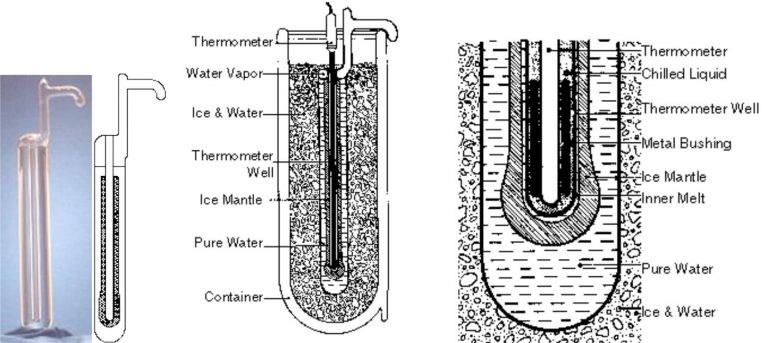Fig. 1. Triple-point-of-water cell, with section and operational details.

To achieve the triple-point state, the cell is kept immersed in an ice-water bath to minimise thermal gradients (or in a thermoelectric controlled bath), and a freezing fluid mixture (e.g. some fine dry-ice chips) is put in the well to force growing an ice mantle around (a few millimetres thick, by viewing the cell from the bottom), without the ice going as far as the outer wall (the ice bridge expansion might brake the cell, some 1000 € worth for a certified cell). Afterwards, a thin layer of this ice mantle is melted next to the well by briefly inserting a rod of aluminium or glass at room temperature into the well, and the cell is ready to work, i.e. the triple point state is reached (for some while, while the ice ring is visible).

Types of thermometers

In the present computer age, thermometers may be grouped in electrical (i.e. which yield an electrical signal, like thermocouples and thermistors), and non-electrical (or mechanical, like mercury and bimetallic), which are seldom used in practice. We describe here the most used thermometers, starting with the old liquid-in-glass familiar type. There are also chromophoric substances (in the shape of sticker labels, pellets, crayons, liquid crystals, and so on), which change colour with temperature variations, either reversibly or irreversibly.

In information process diagrams, standard symbols and labels are used to represent different types of instruments and signal processing. Thermometers are tagged TT (temperature transducer), as pressure transducers are labelled PT, and fluid-flow meters FT.

Liquid-in-glass

Thermal expansion of a substance (solid, liquid or gas) can be used to measure temperature. The coefficient of thermal expansion is roughly α=10-5 1/K for solids, 10-4 1/K for liquids and 10-3 1/K for gases. Bimetal thermometers are based on different expansion of solids, and constant-pressure gas thermometers may also be used. As gases are not easily handed, and solids do not expand so much, liquid-in-glass thermometers are the most common of the expansion type.

A typical liquid-expansion thermometer is shown in Fig. 2; some construction details follow. The bulb is not blown on the capillary tube itself, but is formed of a separate piece of tube fused on the stem. It is possible in this manner to secure greater uniformity of strength and regularity of dimensions. The thickness of the glass is generally between half a millimetre and one millimetre. The advantage shorter response time gained by making the glass thin is more than counterbalanced by increased fragility and liability to distortion. The best form of bulb is cylindrical, of the same external diameter as the stem, to ease insertion through holes. The bore of the stem should also be cylindrical (and not oval or flattened), in order to diminish errors due to capillarity, and to secure the greatest possible uniformity of section.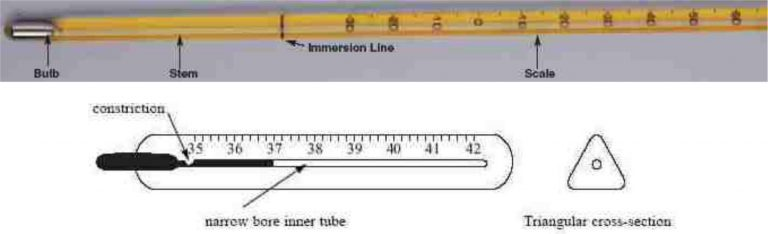Liquid-expansion thermometers are only used to measure temperature in fluids by immersion in them, totally or partially (what has an impact on calibration), but are useless to measure temperature in solids and surfaces. Mercury thermometers have been for the last 400 years the best choice in terms of economical accuracy, although nowadays environmental problems , plus the trend to electronic automation, have relegated them to the museum.

Calibrations of dilatation thermometers are made by comparison with a standard platinum resistance thermometer in a constant temperature bath within the temperature range. Rough calibration with an ice bath and a boiling bath can yield uncertainties of a few tenths of a degree even after pressure corrections.

Thermocouple

A thermocouple is a couple of different conductors electrically connected at one end, the probe tip; between the open ends, a small electrical voltage appears that is proportional to the temperature difference between the joined tip and the open extreme, what is known as Seebeck effect. Thermocouples are the most common temperature sensing devices because of their wide temperature range, smallness, fast, response, toughness, simplicity and economy; provided there are no large thermal gradients along them, inexpensive compensating wires can replace nominal thermocouple conductors outside the sensitive region. Extension cables use the actual thermocouple materials, but in cheaper forms. This is achieved by using cheaper insulations, wider tolerance alloys and thinner conductors (as they will see little thermal stress in their lifetime). Semiconductor materials would yield larger gain than metals, but are too fragile for wires.Some internal details of a thermocouple probe are shown in Fig. 3; PVC, silicon rubber or PTFE are common insulators up to 250 ºC, and glass fibre or ceramic is used above this. The protection sheath is often a metal alloy (or ceramic for high temperatures).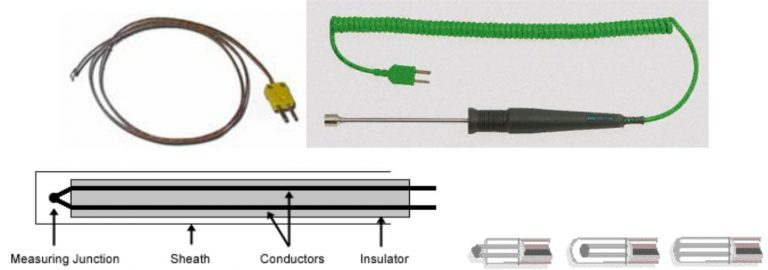Thermocouple with standard connector, surface probe with handle, internal sketch and the three different tip styles for a thermocouple.

Thermocouple output depends on the alloy mix of the conductors. No two mixes will be identical and sensor calibration is required if high accuracy is needed. The performance of an individual sensor will drift with high temperature exposure. Type K thermocouple is the most used, with one wire made of nickel-chromium alloy (‘cromel’) and the other of a nickel-aluminium alloy (‘alumel’), yielding 40 µV/K of temperature difference. With proper sheath, K-thermocouples can measure from −200 ºC to 1300 ºC.

Thermocouples have the fastest response amongst contact thermometers (quantum radiometers can be faster), since micrometre-size wire gauges can be used; e.g. a response time of 2 ms is advertised for 25 µm diameter of bare wires, and there are thinner wires, but not strong enough for practical work). Fine thermocouples are used in hot-gas works to minimise the effect of thermal radiation from the walls, since the convective coefficient, h=Nu·k/d, becomes dominant in the thermal balance of the thermocouple bead, mcdT/dt=hA(Tgas−T)−εAσ(T4T4wall), where d, m, c, T, A, and ε are the bead diameter, mass, thermal capacity, temperature, exposed area, and emissivity, respectively, and k is the fluid thermal conductivity (heat conduction along the wires is negligible with these thin gauges).

Platinum resistance (RTD)

Resistance Temperature Devices (RTD) are based on the change of the electrical resistance of a conductor with temperature. The electrical resistivity of metals can be approximated by ρ=ρ0(1+α(T-T0), with ρ0=0.017 Ω·mm2/m and α=0.0039 1/K for commercial copper, ρ0=0.068 Ω·mm2/m and α=0.007 1/K for nickel, ρ0=0.054 Ω·mm2/m and α=0.0045 1/K for wolfram, and ρ0=0.11 Ω·mm2/m and α=0.003850 1/K for platinum. Platinum is used in most instances because of its wide temperature range, accuracy, linearity, and stability; and Pt-100 probes in particular, where a coiled platinum wire wound around an insulator is used (typically the wire is about 0.05 mm in diameter and 2 m long, with a nominal resistance of 100 Ω at 0°C; the change is thus 0.385 Ω/K). Although no special extension cables or cold-junction compensation is required, RTD have to be powered to work (they are no passive devices like thermocouples), have a small gain, and lead wire resistance should be considered; a three or four wire connection strategy and a Wheatstone bridge are used to eliminate the connection lead resistance effects from measurements.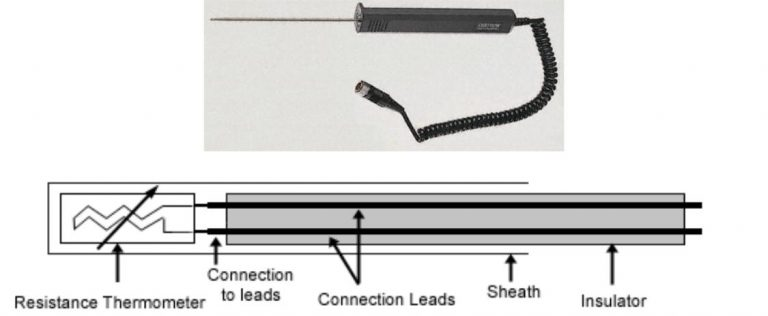The standard platinum resistance thermometer (SPRT) defines the ITS-90 within the range 13.8033 K to 1234.93 K, when calibrated at specific sets of defining fixed points (Table 1 above), and used with specified reference and deviation functions for interpolation at intervening temperatures. Several differently constructed SPRT devices are used to cover the whole range.

Thermistors

Thermistors (Thermal Resistors) are semiconductor materials with a rapidly changing electrical resistance with temperature. Contrary to metal conductors, which always show a positive temperature coefficient for resistivity (α in ρ=ρ0(1+α(T-T0)), most thermistors show a Negative Temperature Coefficient (and are so named NTC-sensors), due to increasing number of electrons in conduction band with temperature (resistance is determined by the density of conduction electrons.). Typical NTC resistance characteristics are R=10 kΩ (±1%) at 25 ºC and dR/dT=−0.5 kΩ/K, instead of the typical RTD values R=100 Ω and dR/dT=0.385 Ω/K. There are also some Positive Temperature Coefficient thermistors (named PTC-sensors), mainly used in electric-current regulation, with a typical resistance of R=1 kΩ (±1%) at 25 ºC (they are made from doped ceramics or polymers). Thermistors are cheap and very sensitive, but they have a relatively narrow range, and require powering like for RTD.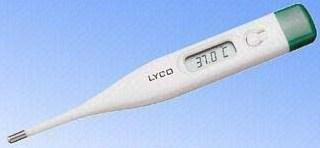Fig. 5. An example of thermistor application: digital clinic thermometer

Integrated Circuit temperature sensors (IC thermometer)

IC temperature sensors are semiconductor devices (two IC transistors coupled in saturated state), which produce an output current proportional to absolute temperature of the device when a voltage is applied (in the typical range 3..30 V DC, the device acts as a high impedance constant current regulator with typically 1 µA/K response). They are cheap and insensible to electromagnetic noise, but their operating range is very narrow (−50 ºC..150 ºC), size cannot be smaller than a few millimetres, and they require powering.High Output. High Accuracy. Inexpensive. CONs: Power Required. Slow. Self Heating. Not meant to be used outdoors.

Diode temperature sensors

Diodes can be used as a temperature measuring device, since the voltage difference between two p-n junctions, operated at different current densities (usually the same current and distinct junction area), is proportional to absolute temperature.

Silicon diode thermometers are most used in cryogenic applications. They have a constant negative thermal coefficient (NTC) of about −2 mV/K between 30 K and 400 K, which amounts to some −25 mV/K below 20 K. Accuracy may be ±0.5 K in the 2..100 K range.

Radiation thermometers and other non-contact thermometers

Non-contact thermometers may employ different physical phenomenon to determine temperature of the tested object: radiation phenomenon, refraction or phase Doppler phenomenon, luminescence phenomenon, Schlieren phenomenon etc. However, almost all systems used in practice for non-contact temperature measurement are based on the reception of thermal radiation from the object, and are termed radiation thermometers (RT). Non-contact temperature measurement is the preferred technique for small, moving, or inaccessible objects; dynamic processes that require fast response; and for high temperatures. Pyrometers are RT for work at high temperatures.

Radiation thermometers can be divided into a few groups according to different criteria: human role in the measurement, location of system spectral bands, presence of an additional cooperating source, number of system spectral bands, number of measurement points, width of system spectral bands and transmission media, etc. Their spectral band is constrained by good transmisivity through the intermediate media (ambient air in most cases), and minimum reflectance from other solids, thus, only portions of the IR spectrum are important, those atmospheric windows that provide maximum loss-free transmission through water vapour and carbon dioxide in air: the near-IR, 0.7..1.3 µm and 1.4..1.8 µm; the mid-IR, 2.0..2.5 µm, 3.2..4.3 µm and 4.8..5.3 µm; and the most used, the far-IR, 8..14 µm. The imaging optics must be transparent to IR radiation (e.g. germanium), and there are two different types of detectors: thermal detectors and quantum detectors. The cheapest IR thermometers have a thermopile detector (i.e. a collection of thermocouples connected to each other in series in order to achieve better temperature sensitivity). IR thermometers have just one sensor, where the radiation from the object is concentrated; i.e. they are of spot or point type. A linear array of sensors, or a 1-D scanning optics can produce a 1-D temperature profile at once, but the most common and practical thermal imaging device is a 2-D array of sensors, or a 2-D scanning optics, or a 2-D sensor electronically scanned like a CCD, producing a temperature map of the region viewed at high sampling rate, i.e. thermal vision (of course, the images displayed can only represent temperature levels in a grey scale or a pseudo-colour arbitrary scale).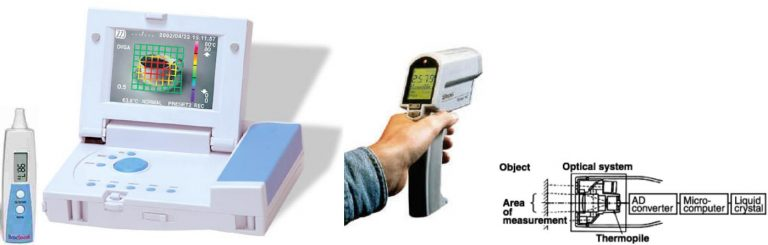Fig. 6. Ear IR-thermometer, 2-D array IR-unit, handheld industrial IR-unit, some internal details.

Comparison among common types of thermometers.

 Characteristic Thermocouple RTD Thermistor Infrared Range −250..2600 °C −200..850 °C −60..300 °C −50..3000 °C Accuracy Less Accurate More Accurate Less Accurate Less Accurate Size Very small, 1 mm Larger, 5 mm Small, 1 mm (non-contact) Linearity Quasi-Linear Quasi-Linear Not Linear Quasi-Linear Reference Junction Required Not Required Not Required Not Required Lead Wire Resistance No Problem Must be Considered No Problem Not Required Response Fast Slower Medium Fast

Specific Heat

Heat Capacity- The heat capacity measures the amount of heat necessary to raise the temperature of an object or system by one degree Celsius.

Heat capacity (usually denoted by a capital C, often with subscripts), or thermal capacity, is the measurable physical quantity that characterizes the amount of heat required to change a substance’s temperature by a given amount. In SI units, heat capacity is expressed in units of joules per kelvin (J/K).

An object’s heat capacity (symbol C) is defined as the ratio of the amount of heat energy transferred to an object to the resulting increase in temperature of the object.

C=QΔT.

Heat capacity is an extensive property, so it scales with the size of the system. A sample containing twice the amount of substance as another sample requires the transfer of twice as much heat (Q) to achieve the same change in temperature (ΔT). For example, if it takes 1,000 J to heat a block of iron, it would take 2,000 J to heat a second block of iron with twice the mass as the first.

### The Measurement of Heat Capacity

The heat capacity of most systems is not a constant. Rather, it depends on the state variables of the thermodynamic system under study. In particular, it is dependent on temperature itself, as well as on the pressure and the volume of the system, and the ways in which pressures and volumes have been allowed to change while the system has passed from one temperature to another. The reason for this is that pressure-volume work done to the system raises its temperature by a mechanism other than heating, while pressure-volume work done by the system absorbs heat without raising the system’s temperature. (The temperature dependence is why the definition a calorie is formally the energy needed to heat 1 g of water from 14.5 to 15.5 °C instead of generally by 1 °C. )

Different measurements of heat capacity can therefore be performed, most commonly at constant pressure and constant volume. The values thus measured are usually subscripted (by p and V, respectively) to indicate the definition. Gases and liquids are typically also measured at constant volume. Measurements under constant pressure produce larger values than those at constant volume because the constant pressure values also include heat energy that is used to do work to expand the substance against the constant pressure as its temperature increases. This difference is particularly notable in gases where values under constant pressure are typically 30% to 66.7% greater than those at constant volume.

### Thermodynamic Relations and Definition of Heat Capacity

The internal energy of a closed system changes either by adding heat to the system or by the system performing work. Recalling the first law of thermodynamics,

dU=δQ−δW.

For work as a result of an increase of the system volume we may write,

dU=δQ−PdV.

If the heat is added at constant volume, then the second term of this relation vanishes and one readily obtains

(∂U∂T)V=(∂Q∂T)V=CV.

This defines the heat capacity at constant volumeCV. Another useful quantity is the heat capacity at constant pressureCP. With the enthalpy of the system given by

H=U+PV,

our equation for dU changes to

dH=δQ+VdP,

and therefore, at constant pressure, we have

(∂H∂T)P=(∂Q∂T)P=CP.

### Specific Heat

The heat capacity is an extensive property that describes how much heat energy it takes to raise the temperature of a given system. However, it would be pretty inconvenient to measure the heat capacity of every unit of matter. What we want is an intensive property that depends only on the type and phase of a substance and can be applied to systems of arbitrary size. This quantity is known as the specific heat capacity (or simply, the specific heat), which is the heat capacity per unit mass of a material. Experiments show that the transferred heat depends on three factors: (1) The change in temperature, (2) the mass of the system, and (3) the substance and phase of the substance. The last two factors are encapsulated in the value of the specific heat.The dependence on temperature change and mass are easily understood. Because the (average) kinetic energy of an atom or molecule is proportional to the absolute temperature, the internal energy of a system is proportional to the absolute temperature and the number of atoms or molecules. Since the transferred heat is equal to the change in the internal energy, the heat is proportional to the mass of the substance and the temperature change. The transferred heat also depends on the substance so that, for example, the heat necessary to raise the temperature is less for alcohol than for water. For the same substance, the transferred heat also depends on the phase (gas, liquid, or solid).

The quantitative relationship between heat transfer and temperature change contains all three factors:

Q=mcΔT

,

where Q is the symbol for heat transfer, m is the mass of the substance, and ΔT is the change in temperature. The symbol c stands for specific heat and depends on the material and phase.

The specific heat is the amount of heat necessary to change the temperature of 1.00 kg of mass by 1.00ºC. The specific heat c is a property of the substance; its SI unit is J/(kg⋅K) or J/(kg⋅C). Recall that the temperature change (ΔT) is the same in units of kelvin and degrees Celsius. Note that the total heat capacity C is simply the product of the specific heat capacity c and the mass of the substance m, i.e.,

C=mc

or

c=Cm=CρV

,

where ϱ is the density of the substance and V is its volume.

Values of specific heat must generally be looked up in tables, because there is no simple way to calculate them. Instead, they are measured empirically. In general, the specific heat also depends on the temperature. The table below lists representative values of specific heat for various substances. Except for gases, the temperature and volume dependence of the specific heat of most substances is weak. The specific heat of water is five times that of glass and ten times that of iron, which means that it takes five times as much heat to raise the temperature of water the same amount as for glass and ten times as much heat to raise the temperature of water as for iron. In fact, water has one of the largest specific heats of any material, which is important for sustaining life on Earth.

.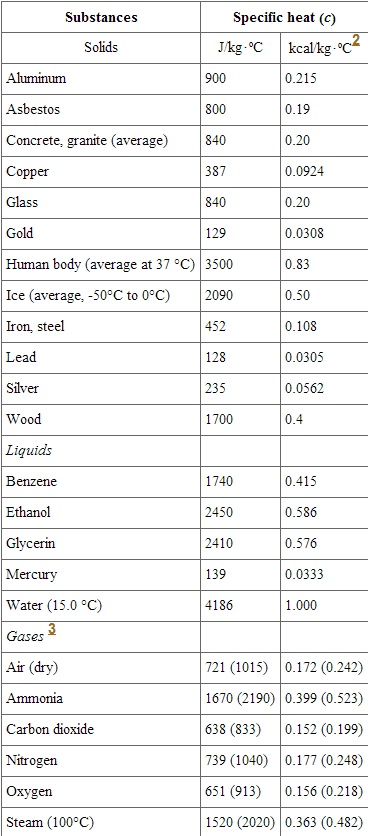### Calorimetry

Calorimetry is the science of measuring the heat of chemical reactions or physical changes. Calorimetry is performed with a calorimeter. A simple calorimeter just consists of a thermometer attached to a metal container full of water suspended above a combustion chamber. The word calorimetry is derived from the Latin word calor, meaning heat. Scottish physician and scientist Joseph Black, who was the first to recognize the distinction between heat and temperature, is said to be the founder of calorimetry.

Calorimetry requires that the material being heated have known thermal properties, i.e. specific heat capacities. The classical rule, recognized by Clausius and by Kelvin, is that the pressure exerted by the calorimetric material is fully and rapidly determined solely by its temperature and volume; this rule is for changes that do not involve phase change, such as melting of ice. There are many materials that do not comply with this rule, and for them, more complex equations are required than those below.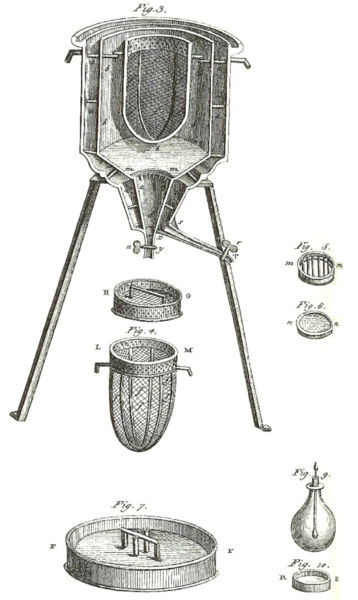Ice Calorimeter: The world’s first ice-calorimeter, used in the winter of 1782-83, by Antoine Lavoisier and Pierre-Simon Laplace, to determine the heat evolved in variouschemical changes; calculations which were based on Joseph Black’s prior discovery of latent heat. These experiments mark the foundation of thermochemistry.

### Basic Calorimetry at Constant Value

Constant-volume calorimetry is calorimetry performed at a constant volume. This involves the use of a constant-volume calorimeter (one type is called a Bomb calorimeter). For constant-volume calorimetry:

δQ=CVΔT=mcVΔT

where δQ is the increment of heat gained by the sample, CV is the heat capacity at constant volume, cv is the specific heat at constant volume, and ΔT is the change in temperature.

### Measuring Enthalpy Change

To find the enthalpy change per mass (or per mole) of a substance A in a reaction between two substances A and B, the substances are added to a calorimeter and the initial and final temperatures (before the reaction started and after it has finished) are noted. Multiplying the temperature change by the mass and specific heat capacities of the substances gives a value for the energy given off or absorbed during the reaction:

Dividing the energy change by how many grams (or moles) of A were present gives its enthalpy change of reaction. This method is used primarily in academic teaching as it describes the theory of calorimetry. It does not account for the heat loss through the container or the heat capacity of the thermometer and container itself. In addition, the object placed inside the calorimeter shows that the objects transferred their heat to the calorimeter and into the liquid, and the heat absorbed by the calorimeter and the liquid is equal to the heat given off by the metals.

Change Of State

Most substances may exist in any of the three common states of matter. In the gaseous state, the molecular motion has completely overcome any attraction between the particles and the particles are totally separate from each other. There are large spaces between the particles and they move large distances between collisions. In the liquid state, the molecular motion and the molecular attractions are more balanced. While the particles stay more or less in contact with each other, they are still free to move and can slide past one another easily. In the solid state, the attractive forces dominate. The particles are pulled together into a tightly packed pattern which does not allow the particles to pass each other. The molecular motion in this form is essentially reduced to vibration in place. Increasing the temperature of a substance means increasing the molecular motion (kinetic energy) of the molecules in the substance. The phase in which a substance exists is the result of a competition between attractive forces and molecular motion.

.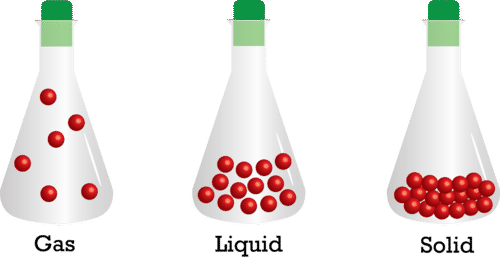For most substances, when the temperature of the solid is raised high enough, the substance changes to a liquid, and when the temperature of the liquid is raised high enough, the substance changes to a gas. We typically visualize a solid as tiny particles in constant motion held together by attractive forces. As we add heat to the solid, the motion, or the kinetic energy, of the particles increases. At some temperature, the motion of the particles becomes great enough to overcome the attractive forces. The thermal energy that was added to the solid up to this point was absorbed by the solid as kinetic energy, increasing the speed of the molecules. The lowest temperature at which the particles are able to exist in the liquid form is called the melting point.

In order for the molecules to actually separate from each other, more energy must be added. This energy, called heat of fusion or heat of melting, is absorbed by the particles as potential energy as the solid changes to a liquid. Recognize that, once the temperature of a solid has been raised to the melting point, it is still necessary for the solid to absorb additional thermal energy in the form of potential energy as the molecules separate.

The boiling point of a liquid is the temperature at which the particles have sufficient molecular motion to exist in the form of a gas. Once again, however, in order for the particles to separate to the gaseous form, they must absorb a sufficient amount of potential energy. The amount of potential energy necessary for a phase change to gaseous form is called the heat of vaporization. Consider the heating curve shown below.The heating curve shown is for water but other substances have similarly shaped heating curves. Suppose you begin with solid water (ice) at -30°C and add heat at a constant rate. The heat you add in the beginning will be absorbed as kinetic energy and the temperature of the solid will increase. When you reach a temperature of 0°C (the melting point for water), the heat you add is no longer absorbed as kinetic energy. Instead, the added heat is absorbed as potential energy and the particles separate from each other. During the flat part of the curve labeled “melting”, heat is being added constantly but the temperature does not increase. At the left edge of this flat line, the water is solid; by the time enough heat has been added to get to the right edge, the water is liquid, but maintains the same temperature. Once all the water is in the liquid form, the added heat will once again be absorbed as kinetic energy and the temperature will increase again. During the time labeled “water being heated as a liquid”, all the added heat is absorbed as kinetic energy.

When a temperature of 100°C (the boiling point of water) is reached, the added heat is once again absorbed as potential energy and the molecules separate from liquid form into gaseous form. When all the substance has been converted into gas, the temperature will again begin to rise. Use the simulation below to further explore the heating curve for water and visualize the how water changes at the molecular level during each phase illustrated in the graph:

 Substance Heat of Fusion, Hf (J/kg)–––––––––– Heat of Vaporization, Hv (J/kg)–––––––––– Copper 2.05×105 5.07×106 Gold 6.30×104 1.64×106 Iron 2.66×105 6.29×106 Methanol 1.09×105 8.78×105 Water 3.34×105 2.26×106

When the temperature of a substance is changing, we can use the specific heat to determine the amount of heat that is being gained or lost. When a substance is changing phase, we can use the heat of fusion or heat of vaporization to determine the amount of heat being gained or lost. When a substance freezes from liquid to solid, the amount of heat given off is exactly the same as the amount of heat absorbed when the substance melts from solid to liquid. The equations for heat gained or lost are given here:

The heat gained or lost during a temperature change: Q=mcΔt.

The heat gained or lost during a phase change of solid to liquid: Q=mHf.

The heat gained or lost during a phase change of liquid to gas: Q=mHv.

## STEM Elearning

We at FAWE have built this platform to aid learners, trainers and mentors get practical help with content, an interactive platform and tools to power their teaching and learning of STEM subjects, more

#### How to find your voice as a woman in Africa

© FAWE, Powered by: Yaaka DN.